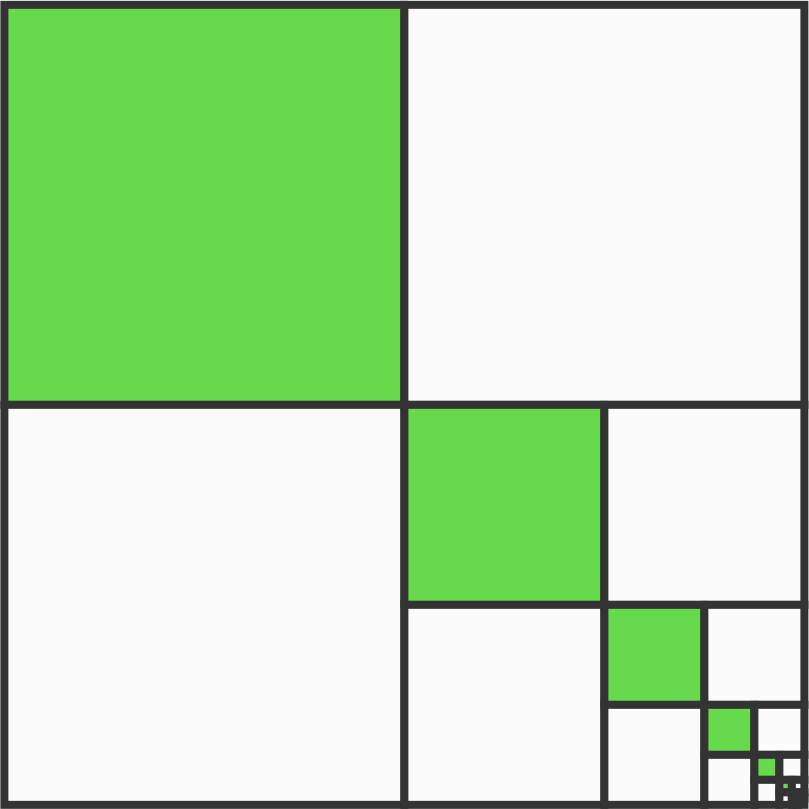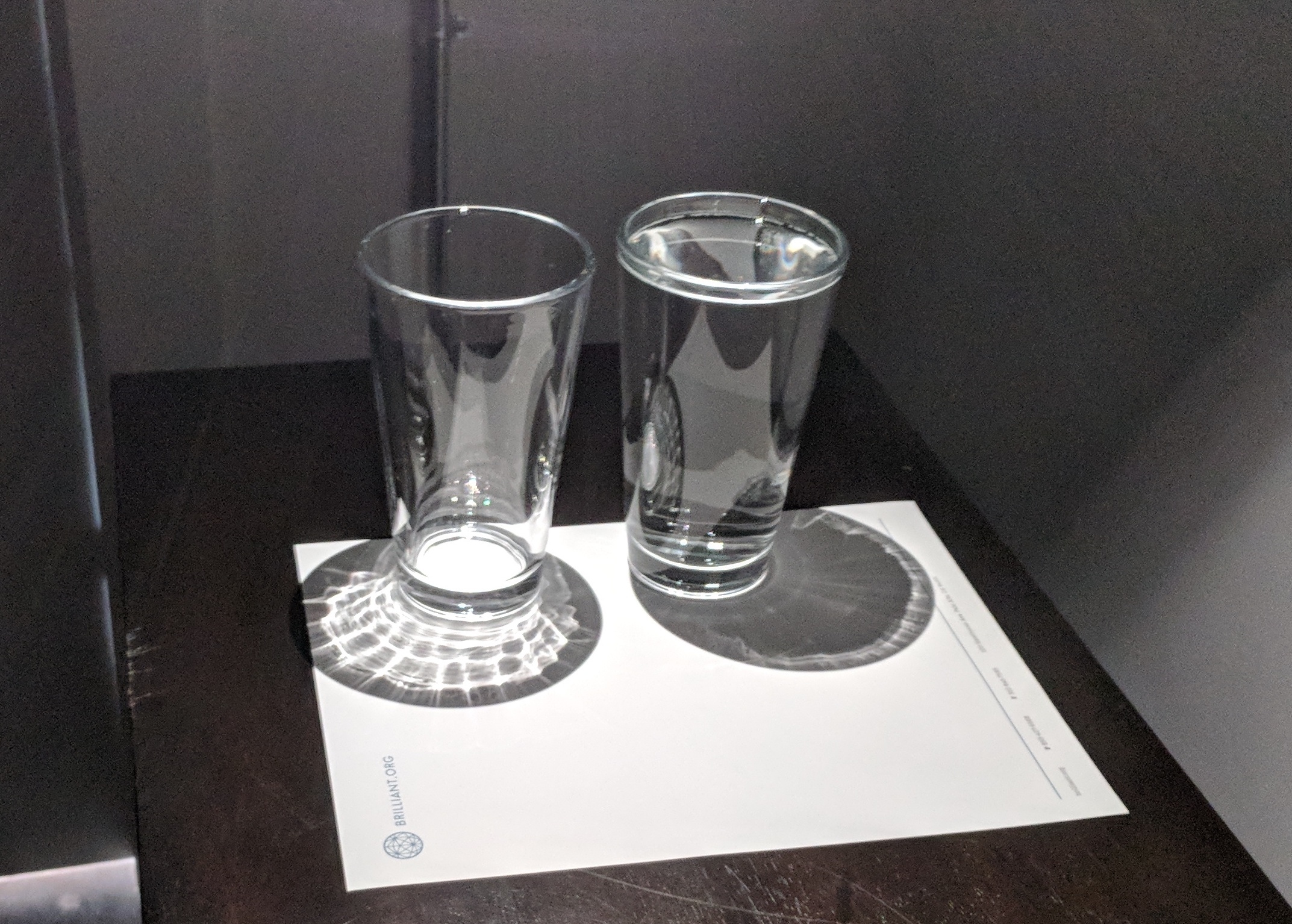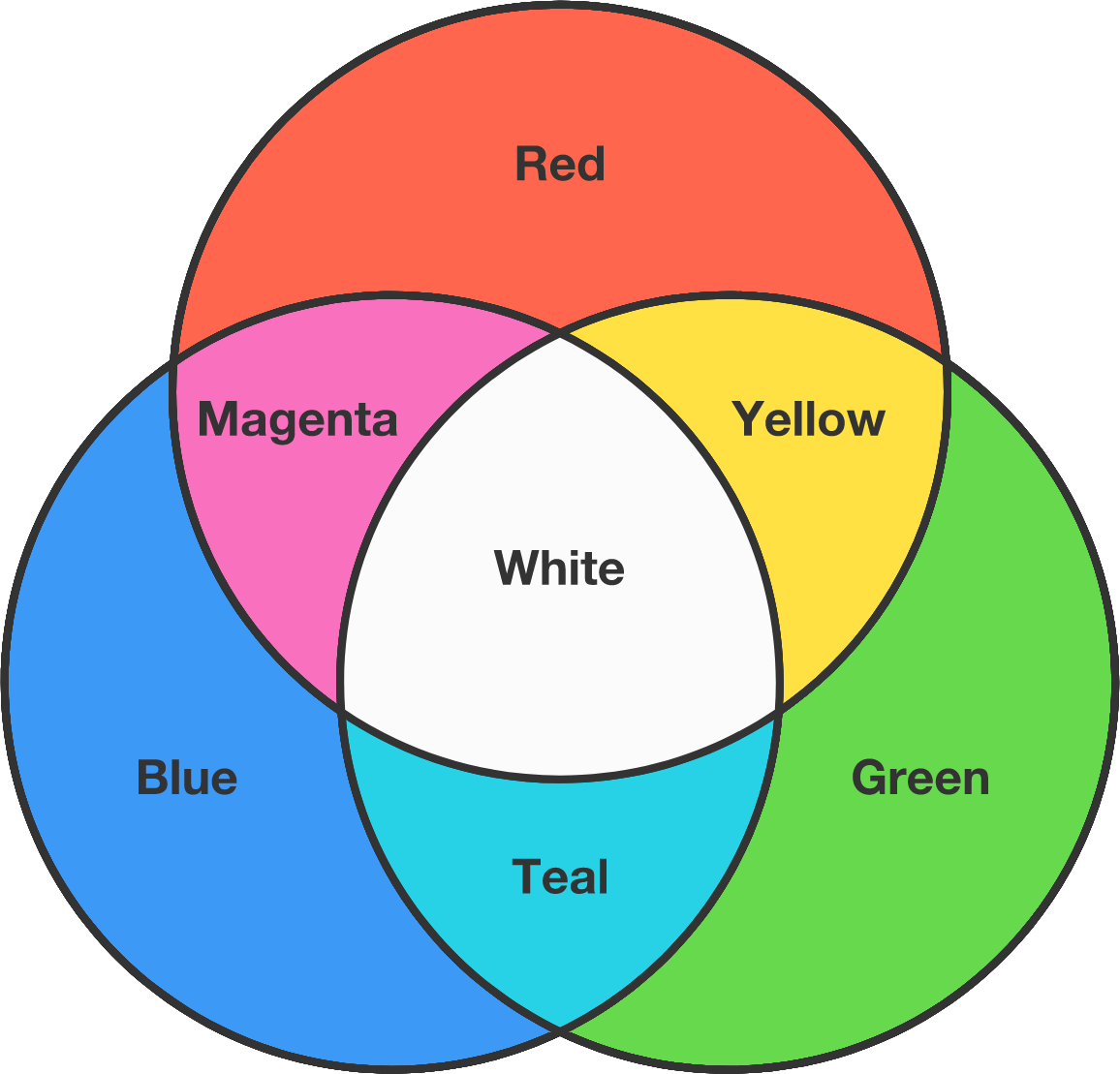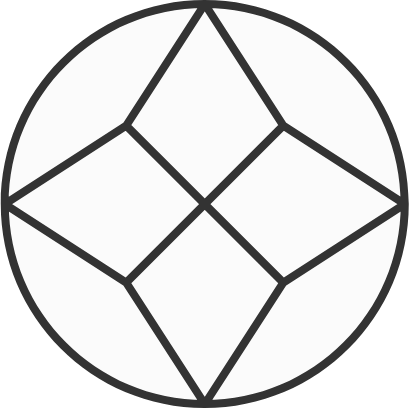# Problems of the Week

Contribute a problem

# 2018-04-23 BasicEvery green square is one quarter of the square it's inscribed in.

If the pattern continues infinitely, what fraction of the total figure will be green?

Why does a full glass of water cast a darker shadow than an empty glass?A blue light shines on a yellow shirt in an otherwise dark room.

What color does the shirt appear?A semiprime is a number that is the product of exactly two (not necessarily distinct) primes. Examples of semiprimes are $6=2 \times 3,$ $35=5 \times 7,$ and $121=11 \times 11$.

It's easy to find pairs $\{21,22\}$ and triplets $\{85,86,87\}$ of consecutive semiprimes on this list of semiprimes up to 187.

Is there a run of consecutive semiprimes longer than three numbers?

Is it possible to cut a circle into pieces with these conditions?

• There are finitely many pieces.
• The pieces must be congruent, meaning that they have the same shape and area.
• Some of the pieces have at most one point on the circumference of the circle.
• Curved cuts are allowed.This cutting is only partially correct. It satisfies all but the "same shape" condition.

×